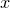## 11.52.80 xlabel

```set <axis>label <text> [ rotate <angle> ]
```

The setting xlabel sets the label which should be written along the x-axis. For example,

```set xlabel '\$x\$'
```

sets the label on the x-axis to read ‘’. Labels can be placed on higher numbered axes by inserting their number after the ‘x’; for example,

```set x10label 'foo'
```

would label the tenth horizontal axis. Similarly, labels can be placed on vertical axes as follows:

```set ylabel '\$y\$'
set y2label 'foo'
```

An optional rotation angle may be specified to rotate axis labels clockwise by arbitrary angles. The angle should be specified either as a dimensionless number of degrees, or as a quantity with physical dimensions of angle.